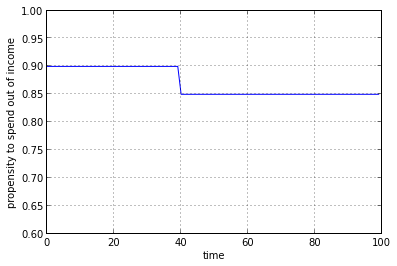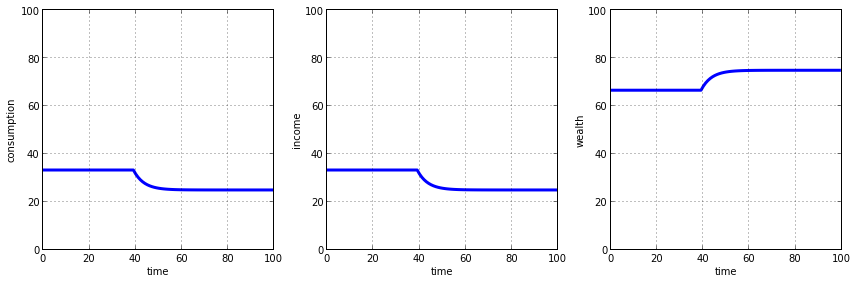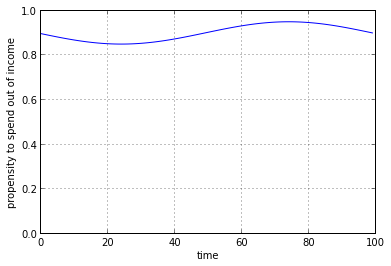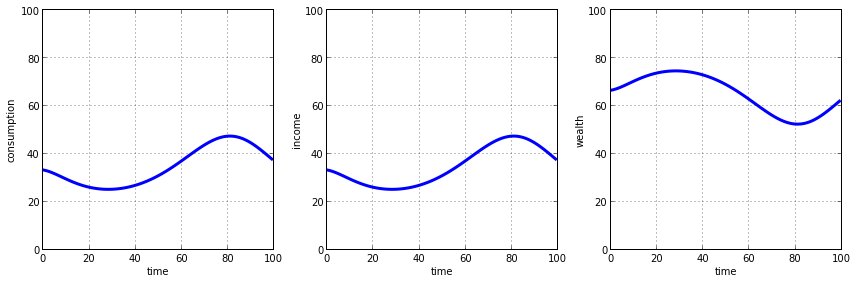# Exogenous and endogenous variables

What does the modeller control and what does the model control?

Posted on 17 July 2016 by Andrew Berkeley

In the previous monetary economics models we set up the scenario we wanted to model, with equations, some numbers for each of the parameters, and some starting ("initial") conditions. Once we set the models up we simply left them to run their course on the basis of the conditions we'd chosen. In this post we'll model a scenario where conditions change during the course of the model run. In doing so we'll draw a distinction between exogenous and endogenous variables.

The variables in any model can be categorized as either exogenous or endogenous. Most simply, exogenous variables are specified outside of the model system and are not changed by the model during the course of its execution. Endogenous variables, on the other hand, are those which are explicitly calculated by the model - they are the values which we want the model to find for us. Exogenous variables, in effect, define the controlled "background" or "forcing" conditions" under which the model is run. The values of endogenous variables which emerge during the model run represent the response of the model to the chosen set of exogenous conditions and the dynamics implied in the model.

In the last model the propensity to spend out of income ($\alpha_Y$) and the propensity to spend out of wealth ($\alpha_H$) were exogenous variables: they were specified at the outset and there was no mechanism inside the model to alter them. The model could not tell us anything about how these variables got their values. They were externally imposed on the model and taken to represent some sort of invariant description of how citizens in the economy choose to spend. The income ($Y$) and spending ($C$) variables were the endogenous variables. Aside from the initial values, we did not know what values $Y$ and $C$ would take at each successive time step in our model - we needed the model to derive these for us. The model therefore solved for the endogenous variables $Y$ and $C$ (and $H$) based on the constraints imposed by the chosen exogenous variables $\alpha_Y$ and $\alpha_H$.

In some cases, exogenous variables may not be variable at all, at least once a particular scenario has been set up by the modeller. The last model, in which constant values for $\alpha_y$ and $\alpha_h$ were used, is a case in point. We might choose to call these values "constants" or "parameters" (as above) instead, so as to make the distinction clear. But there is no law of modelling which states that the modeller must choose a single, constant value for these variables. It might be useful to specify a different value for the propensity to save ($\alpha_y$) at each time step, for example, in order to represent changing saving behaviour through time. Just to generalise even further, if we had a model which resolved spatial dimensions we might want the values of our exogenous variable to vary across space. In both of these cases, the exogenous variable would not be one single value but would take a range of values varying through space and/or time, all specified at the outset by the modeller. So the term "variable" is appropriate for what are external forcing conditions, even if sometimes they happen to be constants. The point of differentiating between exogenous and endogenous variables is to show which variables are assumed by the modeller in setting up the modelling problem and which variables are determined by the dynamics of the model.

# Modelling a time-dependent exogenous variable

Let's take this idea of background-conditions-changing-through-time a little further. We'll take the last model, which represented an economy with spending and saving, and we'll alter the propensity to spend out of income variable ($\alpha_Y$) to vary through time. In mathematical parlance this variable changes from being a constant ($\alpha_Y$) to being a function of time ($\alpha_Y(t)$). We're going to use a very simple function of time: we'll simply change the variable from one value to another part way through the simulation. This (in a highly crude way) can be considered to represent a change in the spending/saving behaviour of our citizens.

Let's set it up. We start in the usual way.

In :
# import required Python libraries
import matplotlib.pyplot as plt
import numpy as np
%matplotlib inline

# set the number of time steps
N = 100

# initialise containers for endogenous variables
C = np.zeros(N) # consumption
Y = np.zeros(N) # income
H = np.zeros(N) # stock of accumulated savings


Now for the $\alpha_Y$ and $\alpha_H$ variables, which previously took constant values of $0.90$ and $0.95$ respectively. This time $\alpha_Y$ will vary through time - we'll start with a value of $0.90$ and reduce it to $0.85$ part way through. Since this variable is now a function of time we need to specify it as an array with a value for every time step. This way, instead of referencing just the the constant value alpha_y as we iterate through time, we can reference the specific value for that time step, alpha_y[t]. The first step is familiar: simply initialise a container - a numpy array - populated with zeros:

In :
alpha_y = np.zeros(N)


Now, we'll set the values that we want. We'll go with the 40th time step for the change in value.

In :
alpha_y[0:40] = 0.90
alpha_y[40:] = 0.85


Let's just make a quick plot to check we have what we wanted.

In :
plt.plot(alpha_y)
plt.ylim([0.6, 1])
plt.grid()
plt.xlabel('time')
plt.ylabel('propensity to spend out of income')

Out:
<matplotlib.text.Text at 0x7f33e87962d0>Okay, we start off with a spending out of income rate of 0.9, which drops to 0.85 at time step 40. Good.

Now let's go with the previous, constant value for the spending out of wealth rate.

In :
alpha_h = 0.05


We need to set our initial conditions. Let's try something else new. When we analysed this model in the last post we found that it settled down to a steady state after about ~30 time steps. We don't want to bother waiting for that to occur - that is not what we're interested in at this stage, we are more interested in how the model responds to our exogenously imposed change. So it would be nice to be able to by pass that initial convergence stage. Well, in the last post we figured out that the $\alpha_Y$ and $\alpha_H$ values used produced steady state savings ($H$) and spending/income ($C$, $Y$) values of $66.66...$ and $33.33...$ respectively. Let's simply start with those values as our initial conditions.

In :
C = 33.33
Y = 33.33
H = 66.66


Okay, we can run the model. Note the reference to alpha_y[t] below, which now has a value for each individual time step and is the only change over the last model.

In :
for t in range(1, N):
C[t] = alpha_y[t] * Y[t-1] + alpha_h * H[t-1]
Y[t] = C[t]
H[t] = H[t-1] + (1 - alpha_y[t]) * Y[t-1] - alpha_h * H[t-1]


And now let's just plot the results (the endogenous variables!).

In :
# create a figure
fig = plt.figure(figsize=(12, 4))

# create a subplot for consumption
# plot consumption (C) versus time step (N)
consumption_plot.plot(range(N), C, lw=3)
consumption_plot.grid()
# ensure a zero origin for the y axis
consumption_plot.set_ylim([0, 100])
# label axes
plt.xlabel('time')
plt.ylabel('consumption')

# create a second subplot for income
# plot income (Y) versus time step (N)
income_plot.plot(range(N), Y, lw=3)
income_plot.grid()
# ensure a zero origin for the y axis
income_plot.set_ylim([0, 100])
# label axes
plt.xlabel('time')
plt.ylabel('income')

# create a third subplot for private wealth
# plot wealth (H) versus time step (N)
wealth_plot.plot(range(N), H, lw=3)
wealth_plot.grid()
# ensure a zero origin for the y axis
wealth_plot.set_ylim([0, 100])
# label axes
plt.xlabel('time')
plt.ylabel('wealth')

# space subplots neatly
plt.tight_layout()Okay we've acheived two things here with respect to the original model. There is no initial "ramp up" period in this model prior to the attainment of steady state. The magnitudes of income and savings start at their steady state, "equilibrium" values and simply plod along at those levels subsequently. That is until our exogenous change occurs. At time step 40, our citizens start spending less of their income - 5% less to be precise. This causes incomes to fall by virtue of the fact that less money is able to flow through the circular flow of spending/incomes. Correspondingly, savings start to rise as the additional residual amount of income that is not spent adds to savings. Eventually, a new steady state level for the economy emerges with income a bit lower and savings a bit higher. Specifically, the new income rate and savings stock equilibrate at the levels associated with the new spending propsensities according to the following expression (see here for derivation):

$\frac{H^*}{Y^*} = \frac{(1 - \alpha_Y)}{\alpha_H}$

i.e the ratio of savings to income is equal to the ratio of the propsentity to save out of income ($1 - \alpha_Y$) to the the propensity to spend out of savings. Previously we had a ratio of:

$\frac {1 - 0.90}{0.05} = \frac {0.10}{0.05} = 2$

so we expected a savings to income ratio of 2. With a fixed money supply of 100, this 2:1 ratio produced a split of 66.6 pounds of savings versus 33.3 pounds of income. Now we have a ratio of:

$\frac {1 - 0.85}{0.05} = \frac {0.15}{0.05} = 3$

and so the new 3:1 ratio between savings and income means that we have 75 pounds in savings and just 25 pounds circulating via spending/income flows.

We've just modelled - in a highly crude way - a recession. Something caused our citizens to save more and consequently spend less. This aggregate attempt to save more caused aggregate incomes to decrease. In this case we do not know what caused our citizens to change track - it was exogenous, meaning that by definition it was determined outside of the model and therefore beyond the explanatory scope of the model. We "forced" the model with this spending behaviour, the model simply showed us what the response in the (simplified) economy would be to such a change.

Let's try a different "forcing" function for $\alpha_Y$...

In :
alpha_y = 0.90 - 0.05*np.sin(np.linspace(1,N,N)*(2*np.pi/N))

plt.plot(alpha_y)
plt.ylim([0, 1])
plt.grid()
plt.xlabel('time')
plt.ylabel('propensity to spend out of income')

Out:
<matplotlib.text.Text at 0x7f33e883ab10>What we've done here is to simply redefine the alpha_y array with new values for each time step. This time we've got a different value at (almost) every time step. We've used a more complicated mathematical function (a sine function) to make the spending behviour of our citizens vary more smoothly through time between $\alpha_Y$ values of $0.85$ and $0.95$.

If we re-run the model with this alpha_y variable, we get:So in this case our citizens immediately begin reining in their spending and this causes aggregate incomes to decrease (and savings to rise). Subsequently, they increase their spending, even beyond the levels seen at the start, and correspondingly aggregate incomes increase (and savings fall). We can view this as a highly contrived "boom-and-bust" cycle. The point, which is illustrated nicely, is that fluctuations in size of the economy ($Y$) can be driven by (cyclical) variations in the spending and saving behaviour of the population. It is worth reiterating that the income and savings curves exactly mirror one another which reflects the fact that the money supply is fixed in this model economy: if savings are to rise, it must come from income; if income is to rise it must be at the expense of savings.

One question that arises from this is: what would cause our citizens to alter their spending and saving habits? Well, anxiety about the (real or perceived) current or expected economic conditions might cause folk and business to be more cautious with their spending and hold back savings as an insurance policy for the future. That is a common interpretation of recessions and economic booms. This would mean that the cause of these fluctuations in spending habits is rooted in the economy itself. So whereas we simply forced this variation in spending behaviour using a completely artifical exogenous spending function, ideally we'd be able to construct a model where the values for $\alpha_Y$ vary dynamically according to what is going on in the model economy at any given point in time. That is, the spending propensities might, in a more sophisticated model, be endogenous.

# Conclusion

Well, we've acknowledged the difference between exogenous and endogenous variables. Exogenous variables are specifed by the modeller and provide background or forcing conditions for driving the model. These forcing conditions might take the form of a function of time if the modeller wishes to understand the response of the model to some sort of change in conditions. Endogenous variables are those calculated by the model - the things we want the model to shed light on for us.

The distinction between exogenous and endogenous variables does, however, depend on the specific way that a model is defined and structured. What is an exogenous variable in one model might be an endogenous variable in another. It largely depends on what the purpose of the model is and what information the modeller has at their disposal.

We've also managed to by-pass the initial transitionary state by setting our initial conditions to known, steady-state values. This is a useful approach when wanting to focus our attention on other aspects of the model solution as it saves computational time and de-clutters the model output.

This post was written using the iPython Notebook

The source can be found on Github or viewed in the iPython Notebook Viewer.

The Python code used in this post is also available in script form on Github here.